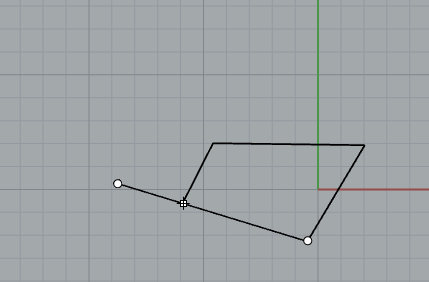# Math in commandline available

Hi,

A lot of time the discussion has come up do evaluate math on the commandline.
Never did I myself had much need for it but today I realized for a project it would come in handy.
What is the status of this? Can we evaluate math like 25+42 in any way as commandline input already?

Thanks
-Willem

``````Command-line calculator
Allows entering mathematical notation on the command line in response to prompts for numbers.
Type =(...) on the command line to evaluate what is inside the parentheses.
The mathematical symbols are
- subtract
* multiply
/ divide
** exponentiation (raise to the power of)
Examples using the Point command:
0,=(3/4+12*2)
=(2.4*(8-2)),=(2.5*2)
=(sqrt(8)-6),0,0
The math module in Python is also supported which means you have access to functions like sin, cos, tan, pi, sqrt, etc. These are listed at http://docs.python.org/library/math.html.``````

Hi Willem - V6 will not require the `=(...)` format. You’ll be able to just type the stuff that currently needs to be inside the brackets in V5.

-Pascal

2 Likes

Hi Willem, if I am working in inches, can I use feet in the command line? Example I am after would be( select line use both sides type 26’/2 and it gives me a line 13’ each side from starting point) while I am asking,can fractions work as well in this manner? Say, 23/32” divided by 2? Thanks ,Mark

Hi Mark,

I have no experience working with fractional inches and feet. So I’ll leave this up to @pascal to answer.

-WIllem

I wish for an option to type “+3” to get the line to be 3 units longer than the snap… would that be possible to implement? Or of course add a “-3” to make it 3 units shorter or even “x3” and “/3” to get multiplications and fractions.
(imagined example:)Hi Holo, does _Subcrv work in the minus as your asking? I haven’t used it enough to know it’s use fully.—-Mark

You could use “From” in this case and type 3 to constrain it to that distance…